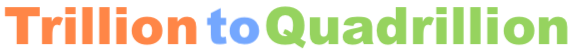Here we will convert 5 trillion to quadrillion. In other words, what is 5 trillion in terms of quadrillion? We start by showing you how to write 5 trillion with numbers:

5,000,000,000,000

There are one thousand trillion in a quadrillion. To give you perspective, below is how to write a quadrillion in numbers.

1,000,000,000,000,000

And here is how our 5 trillion fits into a quadrillion, which will illustrate how much larger a quadrillion is compared to 5 trillion.

1,005,000,000,000,000

Since there are one thousand trillion in a quadrillion, to convert 5 trillion to quadrillion, you would divide 5 trillion by one thousand. Here is the math and the answer:

5 / 1000 = 0.005